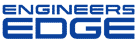# Re: TRUE POSITION ON SPHERE

[ Home ][ Forum Archive ] [ Active Engineering Forum ]

Posted ByKelly Bramble on May 17, 2002 at 20:36:37:

In Reply to: TRUE POSITION ON SPHERE posted byRAY BARBACANO on May 17, 2002 at 15:04:49:

: I was wondering if anybody knew of any documentation on the formula for true position on a sphere.I know the formula for a hole,shaft,etc. is: (x squared plus y squared)square root times 2; I think it would be (x squared plus y squared plus z squared)square root times 3. BUT I DO NOT HAVE ANY DOCUMENTATION?

True position of a sphere is;

Sphere True Position = square root[x squared plus y squared plus z squared]times 2

Sphere Radius = square root[x squared plus y squared plus z squared]

Regards,

< "> Subject: Re: Re: TRUE POSITION ON SPHERE